### MATH 1280 Introduction to Statistics – Midterm Exam – part 5

A painting of Willem de Kooning

Midterm Part 5 – questions 10 - 15, include its answer (total questions are 16)

Use this information for the next six questions:
category <- c('a', 'g', 'd', 'a', 'd', 'g', 'g', 'd')
speed <- c(60, 83.3, 71, 64.4, 75, 90, 87, 73)

10) The data type of the category object is:
Select one:
a. numeric
b. factor …>Correct
c. complex
d. mixed

11) The data type of the speed object is:
Select one:
a. numeric …>Correct
b. factor
c. complex
d. mixed

Another painting of Willem de Kooning

12) What is the mean of the category object?
Select one:
a. 'a'
b. 'd'
c. 'g'
d. between 'd' and 'g'
e. none of the above …>Correct

13) What is the mean of speed (rounded to 1 decimal place)?
Select one:
a. 75.5…> Correct
b. 90.0
c. 60.0
d. 74.0
> speed <- c(60, 83.3, 71, 64.4, 75, 90, 87, 73)
> mean (speed)
Result:
 75.4625

Willem de Kooning’s painting

14) What is the sample standard deviation of the speed data (rounded to 1 decimal place)?
Select one:
a. 113.4
b. 74.5
c. 10.6 …>Correct
d. 90.0
> sd(speed)
Result:
 10.64719

15) What is the minimum value of the speed data:
Select one:
a. 15.1
b. 60.0 …>Correct
c. 74.0
d. 14.9
e. 30.0
> min(speed)
Result:
 60

# To be continued to part 6

1.Interesting quiz :)

1.Thank you to visit:
MATH 1280 Introduction to Statistics – Midterm Exam – part 5
-
A painting of Willem de Kooning

Midterm Part 5 – questions 10 - 15, include its answer (total questions are 16)

Use this information for the next six questions:
category <- c('a', 'g', 'd', 'a', 'd', 'g', 'g', 'd')
speed <- c(60, 83.3, 71, 64.4, 75, 90, 87, 73)

2.MATH 1280 Introduction to Statistics – Midterm Exam – part 5
-
15) What is the minimum value of the speed data:
Select one:
a. 15.1
b. 60.0 …>Correct
c. 74.0
d. 14.9
e. 30.0
> min(speed)
Result:
 60

2.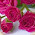Looks interesting!

1.Thank you to visit:
MATH 1280 Introduction to Statistics – Midterm Exam – part 5
-
10) The data type of the category object is:
Select one:
a. numeric
b. factor …>Correct
c. complex
d. mixed

3.I think I failed.

1.Thank you to visit:
MATH 1280 Introduction to Statistics – Midterm Exam – part 5
-
11) The data type of the speed object is:
Select one:
a. numeric …>Correct
b. factor
c. complex
d. mixed

2.MATH 1280 Introduction to Statistics – Midterm Exam – part 5

4.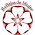1.Thank you to visit:
MATH 1280 Introduction to Statistics – Midterm Exam – part 5
-
12) What is the mean of the category object?
Select one:
a. 'a'
b. 'd'
c. 'g'
d. between 'd' and 'g'
e. none of the above …>Correct

5.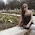Those paintings are so great☺

1.Thank you to visit:
MATH 1280 Introduction to Statistics – Midterm Exam – part 5
-
13) What is the mean of speed (rounded to 1 decimal place)?
Select one:
a. 75.5…> Correct
b. 90.0
c. 60.0
d. 74.0
> speed <- c(60, 83.3, 71, 64.4, 75, 90, 87, 73)
> mean (speed)
Result:
 75.4625

6.I like maths but I must admit that I dont't like Statistics. ;-)

Best regards

1.Thank you to visit:
MATH 1280 Introduction to Statistics – Midterm Exam – part 5
-
14) What is the sample standard deviation of the speed data (rounded to 1 decimal place)?

Select one:
a. 113.4
b. 74.5
c. 10.6 …>Correct
d. 90.0
> sd(speed)

Result:
 10.64719

7.It's all a mystery for me but it sounds interesting. I am not good at math and I am always lost in statistics. But I was fascinated by paintings and love them very much!

1.I see.....

Thank you to visit:
MATH 1280 Introduction to Statistics – Midterm Exam – part 5
-
15) What is the minimum value of the speed data:

Select one:
a. 15.1
b. 60.0 …>Correct
c. 74.0
d. 14.9
e. 30.0

> min(speed)

Result:
 60

8.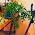nice painting

1.Thank you to visit:
MATH 1280 Introduction to Statistics – Midterm Exam – part 5
-
Use this information for the next six questions:
category <- c('a', 'g', 'd', 'a', 'd', 'g', 'g', 'd')
speed <- c(60, 83.3, 71, 64.4, 75, 90, 87, 73)

9.I was not that good at Maths myself but my daughter was!

1.Thank you to visit:
MATH 1280 Introduction to Statistics – Midterm Exam – part 5
-
10) The data type of the category object is:
Select one:
a. numeric
b. factor …>Correct
c. complex
d. mixed

10.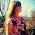Se ven estupendos estos trabajos ! Espero tu opinión en mi última selección de vestidos ! Espero que te gusten ! Feliz día ! ♡♡♡

1.Thank you to visit:
MATH 1280 Introduction to Statistics – Midterm Exam – part 5
-
11) The data type of the speed object is:
Select one:
a. numeric …>Correct
b. factor
c. complex
d. mixed

11.Hello beautiful, a great post, I found it super interesting. Would you like us to follow each other? let me know. a hug

1.Thank you to visit:
MATH 1280 Introduction to Statistics – Midterm Exam – part 5
-
12) What is the mean of the category object?
Select one:
a. 'a'
b. 'd'
c. 'g'
d. between 'd' and 'g'
e. none of the above …>Correct

2.MATH 1280 Introduction to Statistics – Midterm Exam – part 5

12.Interesring, waiting for the next part

1.Thank you to visit:
MATH 1280 Introduction to Statistics – Midterm Exam – part 5
-
13) What is the mean of speed (rounded to 1 decimal place)?
Select one:
a. 75.5…> Correct
b. 90.0
c. 60.0
d. 74.0
> speed <- c(60, 83.3, 71, 64.4, 75, 90, 87, 73)
> mean (speed)
Result:
 75.4625

13.I also failed, but I like the de Kooning paintings!

1.Thank you to visit:
MATH 1280 Introduction to Statistics – Midterm Exam – part 5
-
14) What is the sample standard deviation of the speed data (rounded to 1 decimal place)?

Select one:
a. 113.
b. 74.
c. 10.6 …>Correct
d. 90.0
> sd(speed)

Result:
 10.64719